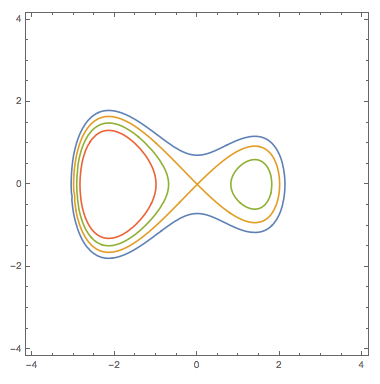# Fact of the day: Functions with given merge tree

$$\def\Real{\mathbb{R}}$$

Consider a Morse function on $latex f:\Real^n\to \Real$ with controlled behavior at infinity, – say, $latex f=|x|^2$ near infinity. Assume further that all critical values $latex a_1<a_2<\ldots<a_k$ are distinct and that all indices of critical points are $latex 0$ or $latex 1$. (Condition obviously holds in one variable.)

Clearly, there are many function that satisfy these conditions. In the (again, most transparent) univariate case, the enumeration of topological types of functions with given critical values is the subject of a nice thread of papers by Arnold on “snakes” (see, e.g., here), relating them to enumeration of ramified coverings of Riemannian spheres, up-down sequences and other cute objects.

We are interested however in a somewhat finer enumeration. Notice that under our index condition, the sublevel sets $latex U(c)=\{f\leq c\}$ for non-critical $latex c$ are collections of topological $latex n$-disks. When increasing $latex c$ crosses a value corresponding to a critical point of index $latex 0$, a new disk is born; when the index is $latex 1$, two disks merge (an alternative would be to generate an element in 1-dimensional homology in the sublevel set for which we don’t have any ammunition – critical points of index 2 – to kill later on).

Combining these births of components and their merges results in a graph which is referred to as “merge tree” and coincides, in dimension $latex n\gt 1$ with the so-called Reeb (a.k.a Reeb-Kronrod) tree.

(Remark: This merge tree completely determines the 0-dimensional persistent diagram corresponding to the filtration by the sublevel sets. The algorithm of decomposing a merge tree into a collection of bars is simple: find the lowest critical point and connect it to the root. The span of the resulting path defines the longest bar. Remove the path and iterate on the resulting subtrees. We note that the bars in those subtrees will be fully contained in the bar corresponding to the removed stem.)

Can one reconstruct the function back from its merge tree? Yes, in the univariate case. Indeed, if the tree is a plane tree, that is if for any merging branches, we know their order left-to-right. In this case, the original function can be obtain using the contour or height walk, called sometimes the Dyck path, see Le Gall’s survey. The height walk will be equivalent (up to reparametrization of the domain) to the original function.

So, how many functions will produce the same merge tree in univariate case: well, the merge tree is binary, and so for each non-leaf vertex we have an option to flip left and right branches, leading to different plane embeddings (at least if all the critical values are distinct) of the same merge tree. Each of them will produce its own height walk, corresponding to the same (up to embedding) merge tree.

Thus, the number of different functions, up to a reparametrization of the domain, resulting in the same merge tree, in univariate case, is $$2^M$$, where $$M$$ is the number of local maxima, i.e. the number of branching vertices in the merge tree. This was observed by Justin Curry.

So, what would be the situation in $$\Real^n$$? It is, actually, very close to the univariate case. Fix a merge tree $$T$$ (a binary tree with distinct real numbers, heights, assigned to its vertices), and denote by $$F(T)$$ the space of Morse functions with merge tree $$T$$. The critical values of these functions correspond to the heights of the merge tree, with exception of the largest one, the root. The critical points of index $$0$$ correspond to the leaves (with exception of the root), and the branching vertices correspond to the critical points of index $$1$$.Theorem:  The space $$F(T)$$ is homotopy equivalent to the product of $$I$$ spheres $$S^{n-1}$$.

The mapping from $$F(T)$$ to $$(S^{n-1})^I$$ is given by the collections of unit vector parallel to the unstable direction of the critical points of index 1, oriented towards the component with deeper minimum.